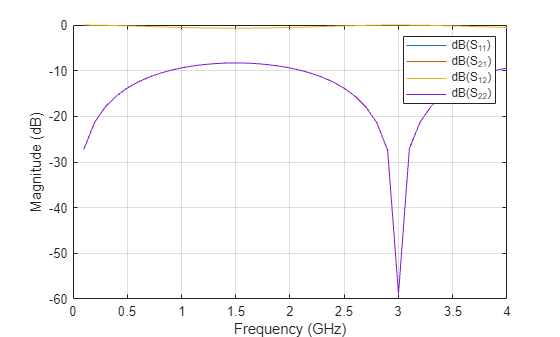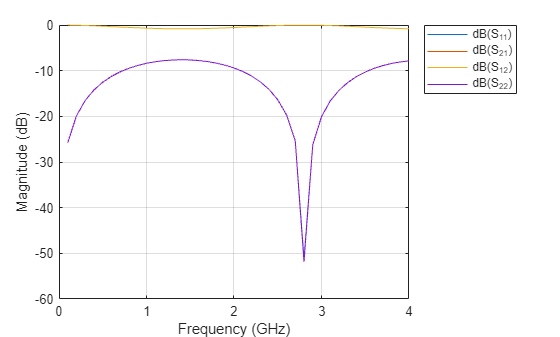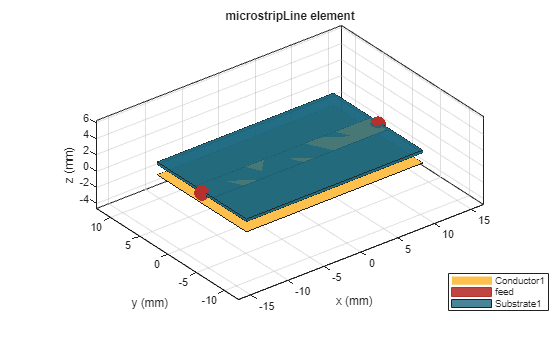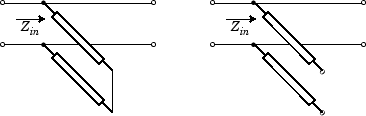# txlineMicrostrip

Create microstrip transmission line

## Description

Use the `txlineMicrostrip` object to create a standard, embedded, inverted, or suspended microstrip transmission line. This figure shows the cross sections of the four types of microstrip transmission lines you can create using the `txlineMircostrip` object. The physical characteristics of the microstrip transmission line include the conductor width (w), the conductor thickness (t), the dielectric thickness (d), the relative permittivity constant (ε), and the height of the conductor above the ground plane (h) .## Creation

### Syntax

``txline = txlineMicrostrip``
``txline = txlineMicrostrip(Name,Value)``

### Description

````txline = txlineMicrostrip` creates a standard microstrip transmission line object.```

example

````txline = txlineMicrostrip(Name,Value)` sets Properties using one or more name-value pairs. For example, ```txline = txlineMicrostrip('Width',0.0046)``` creates a standard microstrip transmission line with a width of `0.0046` meters.```

## Properties

expand all

Name of the microstrip transmission line, specified as a string scalar or a character vector.

Example: `'Name','microstrip1'`

Example: `txline.Name = 'microstrip1'`

Data Types: `char` | `string`

Type of microstrip transmission line, specified as one of the following:

• `'Standard'` Standard microstrip transmission line

• `'Embedded'` Embedded microstrip transmission line

• `'Inverted'` Inverted microstrip transmission line

• `'Suspended'` Suspended microstrip transmission line

Note

When you create a default `txlineMicrostrip` object or set the Type property to 'Standard', the Type property does not show in MATLAB

Example: `'Type','Embedded'`

Example: `txline.Type = 'Embedded'`

Data Types: `char` | `string`

Physical length of the microstrip transmission line, specified as a positive scalar in meters.

Example: `'LineLength',0.0200`

Example: `txline.LineLength = 0.0200`

Data Types: `double`

Physical width of the microstrip transmission line, specified as a positive scalar in meters.

Example: `'Width',0.0008`

Example: `txline.Width = 0.0008`

Data Types: `double`

Physical height of the conductor, specified as a positive scalar in meters.

Example: `'Height',0.000835`

Example: `txline.Height = 0.000835`

Data Types: `double`

Dielectric thickness of the inverted, embedded, or suspended microstrip transmission line, specified as a positive scalar in meters. Default values of dielectric thickness for the embedded, inverted, and suspended microstrip transmission lines are is listed in the table.

Type of MicrostripDefault value of `DielctricThickness`
`'Embedded'``Height`*2
`'Inverted'``Height`
`'Suspended'``Height`/2

Note

• When you create a standard microstrip transmission line, the `DielectricThickness` property does not show in MATLAB.

• By default `txlineMicrostrip` object sets the dielectric thickness of the standard microstrip transmission line to the value of the Height.

Example: `'DielectricThickness',0.0012`

Example: `txline.DielectricThickness = 0.0012`

#### Dependencies

To enable this property, set `Type` as `'Embedded'` or `'Inverted'`, or `'Suspended'`.

Data Types: `double`

Physical thickness of the microstrip transmission line, specified as a positive scalar in meters. You can now model microstrip with thickness set to `0` mm.

Example: `'Thickness',0.000008`

Example: `txline Thickness = 0.000008`

Data Types: `double`

Relative permittivity of the dielectric, specified as a positive scalar.

Example: `'EpsilonR',8.8`

Example: `txline.EpsilonR = 8.8`

Data Types: `double`

Loss angle tangent of the dielectric, specified as a nonegative scalar.

Example: `'LossTangent',1`

Example: `txline.LossTangent = 1`

Data Types: `double`

Conductivity of the conductor, specified as a nonnegative scalar in Siemens per meter (S/m).

Example: `'SigmaCond',2`

Example: `txline.SigmaCond = 2`

Data Types: `double`

Stub transmission line termination, specified as `'NotApplicable'`, `'Open'`, or `'Short'`.

Example: `'Termination','Short'`

Example: `txline.Termination = 'Short'`

Data Types: `char`

Type of stub, specified as `'NotAStub'`, `'Series'`, or `'Shunt'`.

Example: `'StubMode','Series'`

Example: `txline.StubMode = 'Series'`

Data Types: `char`

Number of input and output ports, returned as a positive scalar.

Data Types: `double`

Terminals of the microstrip transmission line, returned as a cell array of strings.

Data Types: `char` | `string`

## Object Functions

 `sparameters` Calculate S-parameters for RF data, network, circuit, and matching network objects `groupdelay` Group delay of S-parameter object or RF filter object or RF Toolbox circuit object `noisefigure` Calculate noise figure of transmission lines, series RLC, and shunt RLC circuits `getZ0` Calculate characteristic impedance with and without dispersion for transmission line `circuit` Circuit object `clone` Create copy of existing circuit element or circuit object

## Examples

collapse all

Create a microstrip transmission line using these specifications:

• Width: `0.08` mm

• Height: `1.6` mm

• Line length: `12.2777` mm

• Thickness: `10e-6` m

• Conductivity: `5.88e7` S/m

• Relative permittivity of the dielectric: `3.9`

```microstriptxline = txlineMicrostrip('Width',0.08e-3,'Height',1.6e-3,... 'LineLength',12.2777e-3,'Thickness',10e-6,'EpsilonR',3.9,'SigmaCond',5.88e7);```

Calculate the S-parameters of the transmission line at `10` GHz.

`sparam = sparameters(microstriptxline,10e9,50);`

Calculate the group delay of the transmission line at `10` GHz.

`gd = groupdelay(microstriptxline,10e9,'Impedance',50)`
```gd = 4.2440e-11 ```

This example uses RF PCB Toolbox to calculate electromagnetic (EM) solver S-parameters of the microstrip line.

Create Suspended Microstrip Line

Create a suspended microstrip transmission line with a copper conductor and Teflon substrate.

```tx = txlineMicrostrip('Type','Suspended',... 'LineLength',0.04705,'Width',3.5e-3,... 'Height',1.6e-3,"DielectricThickness",0.8e-3,... "EpsilonR",2.1,"LossTangent",0.2e-3,... 'SigmaCond',596e5,"Thickness",3.556e-5,... "StubMode","NotAStub","Termination","NotApplicable");```

Behavioral Modeling

Calculate and plot the S-parameters with the reference impedance of `50`$\Omega$.

```freq = (1:40)*100e6; Srf = sparameters(tx,freq,50); rfplot(Srf)```Calculate the characteristic impedance.

`Zc_rf = getZ0(tx)`
```Zc_rf = 75.0279 ```

EM Modeling

Input the microstrip transmission line to the microstripLine object from the RF PCB Toolbox for EM modeling.

`tx_em = microstripLine(tx)`
```tx_em = microstripLine with properties: Length: 0.0471 Width: 0.0035 Height: 0.0016 GroundPlaneWidth: 0.0175 Substrate: [1x1 dielectric] Conductor: [1x1 metal] ```

View the suspended microstrip transmission line.

`show(tx_em)`Calculate and plot the S-parameters using EM solver from RF PCB Toolbox.

```Sem = sparameters(tx_em,freq,50); rfplot(Sem)````Zc_em = getZ0(tx_em)`
```Zc_em = 72.6234 - 0.2018i ```

Select the dielectric and metal layers for an inverted microstrip transmission line from the dielectric and metal libraries, respectively, of the RF PCB Toolbox.

```dFR4 = dielectric('FR4'); dFR4.Thickness = 3.2e-4; mCopper = metal('Copper');```

Create an inverted microstrip transmission line with a copper conductor and an FR4 substrate at 6 GHz with the line length of 0.5$\lambda$ and the reference impedance of 75$\Omega$. The air to substrate thickness ratio is calculated using:

`$\frac{\mathrm{Thickness}\text{\hspace{0.17em}\hspace{0.17em}}\mathrm{of}\text{\hspace{0.17em}}\mathrm{air}\text{\hspace{0.17em}}\mathrm{layer}}{\mathrm{Thickness}\text{\hspace{0.17em}\hspace{0.17em}}\mathrm{of}\text{\hspace{0.17em}}\mathrm{substrate}}=\frac{12.8\mathit{e}-4}{3.2\mathit{e}-4}=4$`

```prototype_behavioral = txlineMicrostrip('Type','Inverted',... 'DielectricThickness',dFR4.Thickness,"EpsilonR",dFR4.EpsilonR, ... 'Height',12.8e-4,"LossTangent",dFR4.LossTangent, ... "SigmaCond",mCopper.Conductivity,"Thickness",mCopper.Thickness);```

Input the inverted microstrip transmission line to the `microstripLine` object from the RF PCB Toolbox for EM modeling.

`prototype_em = microstripLine(prototype_behavioral);`

Use the `design` (Antenna Toolbox) function to design the `microstripLine` (RF PCB Toolbox) object at 6 GHz with the line length of 0.5$\lambda$ and reference impedance of 75$\Omega$.

`tx = design(prototype_em,6e9,'Z0',75,'LineLength',0.5);`

View the `microstipLine` object.

`show(tx)`Plot S-Parameters

Calculate and plot the behavioral and electromagnetic (EM) solver modeled S-parameters of the line with the reference impedance of 50$\Omega$. Use the `Behavioral` name-value argument of the `sparameters` (RF PCB Toolbox) function to compute the behavioral S-parameters.

```freq = (1:5:66)*100e6; Srf = sparameters(tx,freq,50,'Behavioral',true); Sem = sparameters(tx,freq,50); rfplot(Srf,'-s','db') hold on rfplot(Sem,'-x','db') title('Behavioral vs. EM solver modeled S-parameters');```## Algorithms

• When you set the `StubMode` property to `'Shunt'`, the 2-port network consists of a stub transmission line that you can terminate with either a short circuit or an open circuit.Zin is the input impedance of the shunt circuit. The ABCD-parameters for the shunt stub are calculated as:

`$\begin{array}{c}A=1\\ B=0\\ C=1/{Z}_{in}\\ D=1\end{array}$`

When you set the `StubMode` property to `'Series'`, the 2-port network consists of a series transmission line that you can terminate with either a short circuit or an open circuit.Zin is the input impedance of the series circuit. The ABCD-parameters for the series stub are calculated as:

`$\begin{array}{c}A=1\\ B={Z}_{in}\\ C=0\\ D=1\end{array}$`

 Garg, Ramesh, I. J. Bahl, and Maurizio Bozzi. Microstrip Lines and Slotlines . 3rd ed. Artech House Microwave Library. Boston: Artech House, 2013.

 Wadell, Brian C. Transmission Line Design Handbook. The Artech House Microwave Library. Boston: Artech House, 1991.